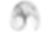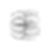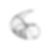# De Jong Attractors

· 3 min readIf you have been following me on Twitter, you would know that I have started experimenting with Generative Art over the last few weeks. While I initially started by just playing with circles and arcs and mixing random values in their equations, I still managed to get some reasonable output with them.

I then came across Strange Attractors and I immediately got hooked. An attractor is a system of numerical values toward which a system tends to evolve even for a variety of initial conditions(I'm quoting straight from Wikipedia). Strange Attractors are a kind of Chaotic System where the chaotic behaviour is found only in a subset of phase space. When the chaotic behavior takes place on an attractor, a large set of initial conditions leads to orbits that converge to this chaotic region. Due to this behaviour, they are often very beautiful to look at.

There are a variety of attractors, like Lorenz Attractor(a post about this is coming soon), De Jong Attractor, Clifford Attractor and Aizawa Attractor.

The De Jong Attractor is defined by the following equations:

xt+1 = sin(a * yt) - cos(b * xt)

yt+1 = sin(c * xt) - cos(d * yt)

where, `a`, `b`, `c` and `d` are the initial conditions.

Generating the attractor with HTML Canvas and JavaScript is straightforward.

``````// 0.2 million points
let n = 200000;

context.translate(width / 2, height / 2);

let previousPoint = { x: 1, y: 1 };

for (let index = 1; index <= n; index++) {
let point = {
x: (Math.sin((a * previousPoint.y)) - Math.cos(b * previousPoint.x)),
y: (Math.sin((c * previousPoint.x)) - Math.cos(d * previousPoint.y))
};

// Scale by 300
context.moveTo(point.x * 300, point.y * 300);
// Draw a point at (x, y)
context.arc(point.x * 300, point.y * 300, 1, 0, 2 * Math.PI);

previousPoint = point;
}

context.stroke();
``````

By changing the value of `a`, `b`, `c` and `d`, different behaviours of the attractor can be generated.

For `a = 2, b = -7, c = -1.2, d = 2`,For `a = -2, b = -2, c = -1.2, d = 2`,If you would like to see more, you can check out the repository on GitHub.

Reference: https://www.algosome.com/articles/strange-attractors-de-jong.html